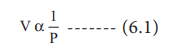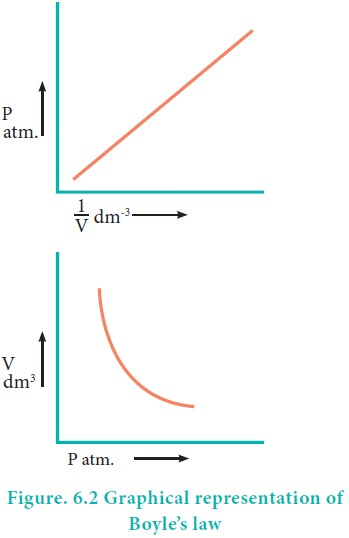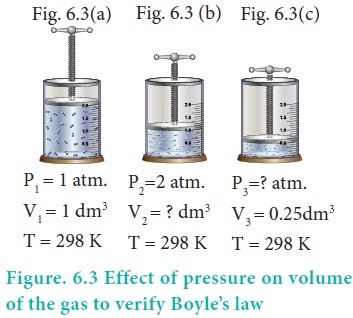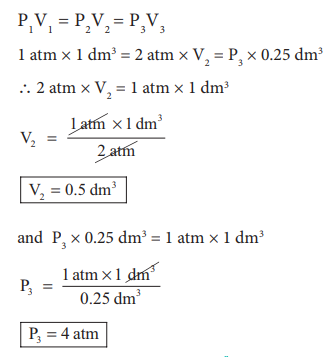Home | | Chemistry 11th std | BoyleŌĆÖs Law: Pressure-Volume Relationship

# BoyleŌĆÖs Law: Pressure-Volume Relationship

Robert Boyle performed a series of experiments to study the relation between the pressure and volume of gases.

BoyleŌĆÖs Law: Pressure-Volume Relationship

Robert Boyle performed a series of experiments to study the relation between the pressure and volume of gases. The schematic of the apparatus used by him is shown in figure 6.1.Mercury was added through the open end of the apparatus such that the mercury level on both ends are equal as shown in the figure 6.1(a). Add more amount of mercury until the volume of the trapped air is reduced to half of its original volume as shown in figure 6.1(b). The pressure exerted on the gas by the addition of excess mercury is given by the difference in mercury levels of the tube. Initially the pressure exerted by the gas is equal to 1 atm as the difference in height of the mercury levels is zero. When the volume is reduced to half, the difference in mercury levels increases to 760 mm. Now the pressure exerted by the gas is equal to 2 atm. It led him to conclude that at a given temperature the volume occupied by a fixed mass of a gas is inversely proportional to its pressure.

Mathematically, the BoyleŌĆÖs law can be written as(T  and n  are  fixed,  T-temperature, n- number of moles)k ŌĆō proportionality constant

When we rearrange equation 6.2.

PV = k at constant temperature and mass

BoyleŌĆÖs law is applicable to all gases regardless of their chemical identity (provided the pressure is low). Therefore, for a given mass of a gas under two different sets of conditions at constant temperature we can write

P1V1 = P2V2  = k  ------(6.3)The PV relationship can be understood as follows. The pressure is due to the force of the gas particles on the walls of the container. If a given amount of gas is compressed to half of its volume, the density is doubled and the number of particles hitting the unit area of the container will be doubled. Hence, the pressure would increase twofold.

Consequence of BoyleŌĆÖs law

The pressure-density relationship can be derived from the BoyleŌĆÖs law as shown below.

P1V1 = P2V2   (BoyleŌĆÖs law)where ŌĆ£mŌĆØ is the mass, d1 and d2 are the densities of gases at pressure P1 and P2.In other words, the density of a gas is directly proportional to pressure.

In figure (6.3) let us find the missing parameters (volume in 6.3 (b) and pressure in 6.3(c))Solution:

According to BoyleŌĆÖs law, at constant temperature for a given mass of gas at constant temperature,

P1V1 = P2V2 = P3V3

1 atm ├Ś 1 dm3 = 2 atm ├Ś V2 = P3 ├Ś 0.25 dm3

Ōł┤ 2 atm ├Ś V2 = 1 atm ├Ś 1 dm3P3 = 4 atm

### Allthe passenger aeroplane cabins have to be artificially pressurised. do you know why?

Most commercial aeroplanes fly at about 30,000 feet altitude. The pressure decreases with the increase in altitude as there are fewer molecules per unit volume of air. Hence, while at air, the pressure around the aeroplane will be so low that one could pass out for lack of oxygen. For this reason aeroplanes cabins are artificially pressurized.Similarly, the effect of drop in pressure is felt as a little pain in the ears by a person while ascending a mountain in a plain. Though the external pressure drops, the internal pressure within the ear cavities remains the same. This creates an imbalance. The greater internal pressure forces the eardrum to bulge outward causing pain. The excess air within the ear cavities escapes after some time and with the help of yawning and thereby equalizing the internal and external pressure to relieve the pain.

### Underwater divers are advised not to hold the breath unnecessarily while diving. Do you know why?

Tags : The Gas Laws , 11th Chemistry : UNIT 6 : Gaseous State
Study Material, Lecturing Notes, Assignment, Reference, Wiki description explanation, brief detail
11th Chemistry : UNIT 6 : Gaseous State : BoyleŌĆÖs Law: Pressure-Volume Relationship | The Gas Laws